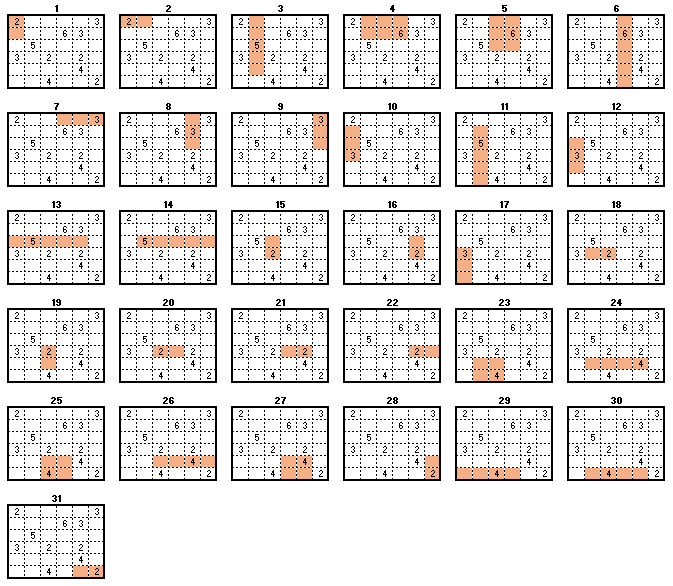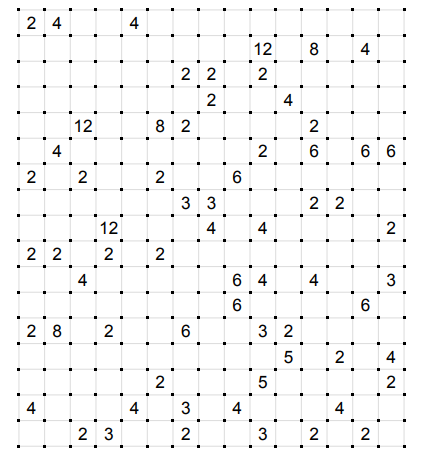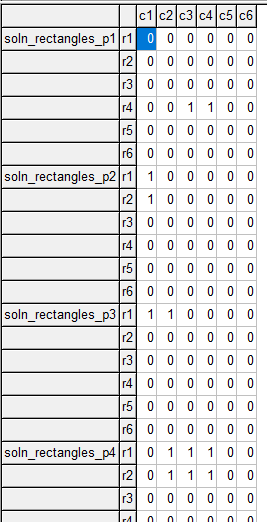## Thursday, March 12, 2020

### CellBlock or Shikaku puzzle

This is a simple puzzle: given a grid with some cells containing a positive integer, form rectangles such that

• Each rectangle contains exactly one cell with a number
• The size of the rectangle is equal to this number
• Every cell in the grid is covered by exactly one rectangle
Example:Shikaku Problem and Solution

#### Approach

One possible approach is a two-step algorithm:

1. Generate all feasible rectangles,
2. Use a simple MIP model to select a subset of these rectangles such that each cell is covered exactly once.

For the problem above, we just have 31 feasible rectangles. Feasible means here: (a) rectangle contains exactly one cell with a number, (b) the size of the rectangle is equal to that number.All feasible rectangles

Designing an algorithm that generates all feasible rectangles is easier said than done, especially if you want to do this efficiently for larger instances. This is mainly a programming exercise, rather than a modeling problem.

The second part is surprisingly simple. Assume all $$k\in K$$ rectangles are stored in a single large boolean data structure: $\mathit{allRectangles}_{k,i,j} = \begin{cases} 1 & \text{if cell $$(i,j)$$ is part of rectangle $$k$$}\\ 0 & \text{otherwise}\end{cases}$ then the optimization model is a simple set covering model:

Set Covering Problem
\begin{align}\min\>& 0\\ &\sum_k \color{darkblue}{\mathit{allRectangles}}_{k,i,j} \color{darkred}x_{k} = 1&& \forall i,j\\ &\color{darkred}x_{k} \in \{0,1\} \end{align}

In this case, we don't have an objective. We simply state that every cell $$(i,j)$$ must be covered by exactly one rectangle.

This is a tiny model and solves very easily. Typically it can be solved completely in the presolve phase:

Tried aggregator 1 time.
MIP Presolve eliminated 37 rows and 32 columns.
MIP Presolve modified 3 coefficients.
All rows and columns eliminated.


The solution can look like:

----     89 VARIABLE x.L  selection of rectangles

k1  1.000,    k3  1.000,    k5  1.000,    k8  1.000,    k9  1.000,    k12 1.000,    k20 1.000,    k22 1.000
k26 1.000,    k29 1.000,    k31 1.000

----     97 PARAMETER result  solution: selected rectangles

c1          c2          c3          c4          c5          c6

r1           1           3           5           5           8           9
r2           1           3           5           5           8           9
r3          12           3           5           5           8           9
r4          12           3          20          20          22          22
r5          12           3          26          26          26          26
r6          29          29          29          29          31          31


The model selects 11 rectangles. We could have predicted this: there are 11 numbered cells in the input data. The parameter result displays the selected rectangle numbers on the grid. You should be able to match this up with the picture of the feasible rectangles shown earlier.

#### GAMS Model

 $ontext Cell Block or Shikaku puzzle Set Covering model for (small) square grids erwin@amsterdamoptimization.com$offtext sets   i 'rows' /r1*r6/   j 'columns' /c1*c6/ ; scalars    m 'rows'    n 'columns' ; m = card(i); n = card(j); alias(i,p,h); alias(j,q,w); table grid(i,j)      c1 c2 c3 c4 c5 c6 r1    2              3 r2             6  3 r3       5 r4    3     2     2 r5                4 r6          4        2 ; scalar nn 'sum of numbers'; nn = sum((i,j),grid(i,j)); display nn; abort$(nn <> card(i)*card(j)) "error in grid specification"; *------------------------------------------ * generate all possible rectangles * requirements: * 1. block contains exactly one number from grid * 2. size of block is equal to this number *------------------------------------------ set k 'rectangle number' /k1*k10000/ allRectangles(k,i,j) r(i,j) 'current rectangle' ; singleton set kk(k) 'current index' /k1/ ; loop((p,q), loop((w,h)$(ord(p)+ord(h)-1<=m and ord(q)+ord(w)-1<=n), * candidate rectangle       r(i,j) = (ord(i)>=ord(p) and ord(i)<=ord(p)+ord(h)-1 and                 ord(j)>=ord(q) and ord(j)<=ord(q)+ord(w)-1); * check: only one grid number and size = gridnumber       if(sum(r$grid(r),1)=1 and card(r)=sum(r,grid(r)), allRectangles(kk,r) = yes; kk(k)=kk(k-1); ); ); ); *------------------------------------------ * model: select rectangles such that each * cell is covered exactly once *------------------------------------------ binary variable x(k) 'selection of rectangles'; variable z 'dummy objective'; equations dummy 'dummy objective' covering(i,j) 'each cell is covered by exactly one rectangle' ; covering(i,j).. sum(allRectangles(k,i,j),x(k)) =e= 1; dummy.. z =e= 0; model cover /all/; * just need feasibility solve cover minimizing z using mip; display x.l; * reporting parameter result(i,j) 'solution: selected rectangles'; loop(k$(x.l(k)>0.5),    result(i,j)\$allRectangles(k,i,j) = ord(k); ); option result:0; display result;

Notes:
• The data check makes sure the sum of the numbers is equal to the size (area) of the grid.
• The enumeration of the feasible rectangles is using a simplistic and crude algorithm. It may need to be refined for larger instances.
• allRectangles(k,i,j) is implemented as a set.
• As we are looking for a feasible solution only, there is no need to specify a gap with the optcr option.

#### A larger problem

A larger problem is taken from .17 x 15 problem

Some statistics:
• This problem has 17 rows and 15 columns.
• The number of feasible rectangles is 253.
• The number of numbered cells is 66. This is also the number of rectangles we need to select.
• If one wants to make a big impression, mention that the number of ways we can choose 66 out of 253 is: \begin{align} {253 \choose 66} &= 66 281 207 211 442 081 043 167 193 447 008 690 678 844 358 496 354 619 442 275 550 \\ &\approx 6.6 \times 10^{61}\end{align}
The results look like:

----    105 VARIABLE x.L  selection of rectangles

k1   1.000,    k3   1.000,    k6   1.000,    k11  1.000,    k13  1.000,    k16  1.000,    k30  1.000,    k34  1.000
k36  1.000,    k37  1.000,    k41  1.000,    k44  1.000,    k45  1.000,    k51  1.000,    k54  1.000,    k63  1.000
k65  1.000,    k69  1.000,    k73  1.000,    k82  1.000,    k84  1.000,    k87  1.000,    k90  1.000,    k91  1.000
k94  1.000,    k103 1.000,    k107 1.000,    k110 1.000,    k112 1.000,    k125 1.000,    k128 1.000,    k130 1.000
k132 1.000,    k134 1.000,    k136 1.000,    k140 1.000,    k143 1.000,    k150 1.000,    k152 1.000,    k154 1.000
k159 1.000,    k162 1.000,    k171 1.000,    k174 1.000,    k190 1.000,    k192 1.000,    k195 1.000,    k199 1.000
k201 1.000,    k202 1.000,    k203 1.000,    k204 1.000,    k209 1.000,    k216 1.000,    k219 1.000,    k222 1.000
k226 1.000,    k228 1.000,    k235 1.000,    k238 1.000,    k244 1.000,    k245 1.000,    k247 1.000,    k249 1.000
k251 1.000,    k253 1.000

----    113 PARAMETER result  solution: selected rectangles

c1          c2          c3          c4          c5          c6          c7          c8          c9

r1            1           3           3           6           6          11          11          11          11
r2            1           3           3           6           6          11          11          11          11
r3           30          30          30          30          34          34          36          37          37
r4           30          30          30          30          34          34          36          51          51
r5           30          30          30          30          34          34          63          65          65
r6           73          73          73          73          34          34          63          65          65
r7           87          87          90          90          91          91          94          65          65
r8          103         103         103         103         103         103          94         107         107
r9          103         103         103         103         103         103          94         125         125
r10         134         136         136         140         140         143         143         125         125
r11         134         154         154         159         159         159         159         159         159
r12         171         154         154         174         174         174         174         174         174
r13         171         190         190         192         195         195         195         199         199
r14         204         190         190         192         195         195         195         209         209
r15         204         190         190         216         216         219         219         222         222
r16         204         190         190         216         216         235         235         235         238
r17         204         244         244         245         245         245         247         247         249

+         c10         c11         c12         c13         c14         c15

r1           11          11          13          13          16          16
r2           11          11          13          13          16          16
r3           41          41          13          13          44          45
r4           54          54          13          13          44          45
r5           54          54          69          69          44          45
r6           82          84          84          84          44          45
r7           82          84          84          84          44          45
r8          107         110         110         112          44          45
r9          128         128         130         112         132         132
r10         128         128         130         150         150         152
r11         162         162         130         150         150         152
r12         162         162         130         150         150         152
r13         199         201         201         202         203         203
r14         209         209         209         202         203         203
r15         222         222         222         226         226         228
r16         238         238         238         226         226         228
r17         249         249         251         251         253         253


Again the presolver could eliminate all rows and columns.

#### Questions

1. How would a better algorithm that enumerates all feasible rectangles look like? I guess it would start with the cells containing numbers.
2. Can we use the solution pool to automate this step?
3. Is the solution unique?
4. Are there cases where the presolver is not solving the complete problem?
5. Nerdy: when disabling the presolver. it looks like we can solve the problem as an LP. I.e. the binary variables are integer-valued automatically. Is this true?

#### Q2: Solution Pool to generate feasible rectangles

In the comments, a MIP model is proposed that will deliver feasible rectangles. It is worthwhile to reproduce it here. A high-level version of this model can look like:

High-level Feasible Rectangle Model
\begin{align}\min\>& 0 && && \text{Dummy objective}\\ &\sum_{i,j|\color{darkblue}{\mathit{Grid}}_{i,j}} \color{darkred}{\mathit{useCell}}_{i,j} = 1 && && \text{One numbered cell in rectangle}\\ & \sum_{i,j} \color{darkblue}{\mathit{Grid}}_{i,j} \color{darkred}{\mathit{useCell}}_{i,j} = \sum_{i,j} \color{darkred}{\mathit{useCell}}_{i,j} &&&&\text{Number equal to area of rectangle} \\ & \color{darkred}{\mathit{useCell}}_{i,j} = \color{darkred}{\mathit{useRow}}_i \cdot \color{darkred}{\mathit{useColumn}}_j &&\forall i,j && \text{Interior of rectangle} \\ & \color{darkred}{\mathit{useRow}}_i \ge \color{darkred}{\mathit{useRow}}_{i1} \cdot \color{darkred}{\mathit{useRow}}_{i2} && \forall i1 \lt i \lt i2 && \text{Contiguous rows} \\ & \color{darkred}{\mathit{useColumn}}_j \ge \color{darkred}{\mathit{useColumn}}_{j1} \cdot \color{darkred}{\mathit{useColumn}}_{j2} && \forall j1 \lt j \lt j2 && \text{Contiguous columns}\\ & \color{darkred}{\mathit{useCell}}_{i,j},\color{darkred}{\mathit{useRow}}_i,\color{darkred}{\mathit{useColumn}}_j \in \{0,1\} \end{align}

This model is nonlinear: there are quadratic terms. We are lucky, however. The quadratic terms involve only binary variables. This means that we can use a standard linearization:$z=x\cdot y \iff \begin{cases} z \le x \\ z \le y \\ z \ge x+y-1 \end{cases}$ We assume here that $$x,y,x$$ are binary variables. When we apply this, the linearized model looks like:

Linearized Feasible Rectangle Model
\begin{align}\min\>& 0 \\ &\sum_{i,j|\color{darkblue}{\mathit{Grid}}_{i,j}} \color{darkred}{\mathit{useCell}}_{i,j} = 1 \\ & \sum_{i,j} \color{darkblue}{\mathit{Grid}}_{i,j} \color{darkred}{\mathit{useCell}}_{i,j} = \sum_{i,j} \color{darkred}{\mathit{useCell}}_{i,j} \\ & \color{darkred}{\mathit{useCell}}_{i,j} \le \color{darkred}{\mathit{useRow}}_i &&\forall i,j \\ & \color{darkred}{\mathit{useCell}}_{i,j} \le \color{darkred}{\mathit{useColumn}}_j &&\forall i,j \\ & \color{darkred}{\mathit{useCell}}_{i,j} \ge \color{darkred}{\mathit{useRow}}_i + \color{darkred}{\mathit{useColumn}}_j - 1 &&\forall i,j \\ & \color{darkred}{\mathit{useRow}}_i \ge \color{darkred}{\mathit{useRow}}_{i1} + \color{darkred}{\mathit{useRow}}_{i2} - 1 && \forall i1 \lt i \lt i2 \\ & \color{darkred}{\mathit{useColumn}}_j \ge \color{darkred}{\mathit{useColumn}}_{j1} + \color{darkred}{\mathit{useColumn}}_{j2} - 1 && \forall j1 \lt j \lt j2 \\ & \color{darkred}{\mathit{useCell}}_{i,j},\color{darkred}{\mathit{useRow}}_i,\color{darkred}{\mathit{useColumn}}_j \in \{0,1\} \end{align}

After using a solution pool algorithm (this is part of solvers like Cplex and Gurobi) on this model, we get an enumeration of all feasible rectangles:Feasible rectangles
For data set 1, we get 31 rectangles and for the second data set, we get 253 rectangles.

#### Q3. Uniqueness

To establish whether solutions are unique, we can use a standard approach:

1. Solve the optimization problem. Record the optimal values as $$x_k^*$$.
2. Add the cut: $\sum_k x_k^* x_k - \sum_k (1-x_k^*)x_k \le \sum_k x_k^* - 1$ to the problem. This cut forbids the solution $$x_k^*$$ but allows any other solution.
3. Resolve. If infeasible then the solution $$x_k^*$$ is unique.

The cut can be specialized. Let $$M$$ be the number of numbered cells. We know that $\sum_k x_k = M$ With this our cut can look like: $\sum_k x_k^* x_k \le M-1$

An alternative, somewhat heavy-handed approach would be to use the solution pool to prove uniqueness.

When we try this, we see that both data sets yield unique solutions.

#### References

1.Here's an ILP model whose feasible solutions correspond to feasible rectangles. I used it to confirm your counts of 31 and 253.

var UseCell {CELLS} binary;
var UseRow {ROWS} binary;
var UseCol {COLS} binary;

con OneClueCell:
sum { in CLUE_CELLS} UseCell[i,j] = 1;
con CellCount:
sum { in CLUE_CELLS} a[i,j] * UseCell[i,j] = sum { in CELLS} UseCell[i,j];
con CellImpliesRow { in CELLS}:
UseCell[i,j] <= UseRow[i];
con CellImpliesCol { in CELLS}:
UseCell[i,j] <= UseCol[j];
con RowAndColImplyCell { in CELLS}:
UseRow[i] + UseCol[j] - 1 <= UseCell[i,j];
con RowContiguity {i1 in ROWS, i2 in ROWS, i3 in ROWS: i1 < i2 < i3}:
UseRow[i1] + UseRow[i3] - 1 <= UseRow[i2];
con ColContiguity {j1 in COLS, j2 in COLS, j3 in COLS: j1 < j2 < j3}:
UseCol[j1] + UseCol[j3] - 1 <= UseCol[j2];

2.Looks like my angle brackets were treated as markup. I hope you can fill in the missing i,j indexers.

3.Regarding question 3, it depends on the grid layout. There could be autonomous regions where an alternate assignment exists, like for example a 2x2 square with empty diagonal and another with 2: ([2,0],[0,2]). If we consider empty cells as a supply pool and cells with numbers as demand centers, then the puzzle become a network flow maximization problem. When I looked at puzzle, I try to identify empty cells that are reachable only from a single demand center. one hard topic there is how to select a region of interest and test it for supply-demand balance.
Thank you for bringing this topic, I always like to read your blog for ideas being discussed.

4.Two minor corrections to your latest update: 1. In the high-level model, the contiguity constraints should both be >= instead of =. 2. x,y,x->x,y,z

5.One more: z \ge x + y = 1 should be z \ge x + y - 1

1.Yep, thanks.